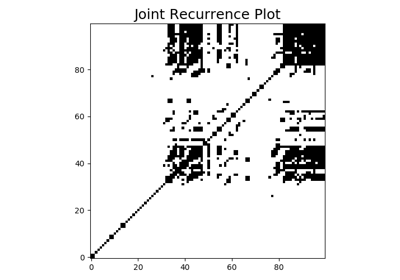# `pyts.multivariate.image`.JointRecurrencePlot¶

class `pyts.multivariate.image.``JointRecurrencePlot`(dimension=1, time_delay=1, threshold=None, percentage=10)[source]

Joint Recurrence Plot.

A recurrence plot is an image representing the distances between trajectories extracted from the original time series.

A joint recurrence plot is an extension of recurrence plots for multivariate time series: it is the Hadamard of the recurrence plots obtained for each feature of the multivariate time series.

Parameters: dimension : int or float (default = 1) Dimension of the trajectory. If float, it represents a percentage of the size of each time series and must be between 0 and 1. time_delay : int or float (default = 1) Time gap between two back-to-back points of the trajectory. If float, it represents a percentage of the size of each time series and must be between 0 and 1. threshold : float, ‘point’, ‘distance’ or None or list thereof (default = None) Threshold for the minimum distance. If None, the recurrence plots are not binarized. If `threshold='point'`, the threshold is computed such as `percentage` percents of the points are smaller than the threshold. If `threshold='distance'`, the threshold is computed as the `percentage` of the maximum distance. percentage : int, float or list thereof (default = 10) Percentage of black points if `threshold='point'` or percentage of maximum distance for threshold if `threshold='distance'`. Ignored if `threshold` is a float or None. Note that the percentage is calculated for each recurrence plot independently, which implies that there will probably be less than percentage percents of black points in the joint recurrence plot.

References

 [R0517172527b3-1] M. Romano, M. Thiel, J. Kurths and W. con Bloh, “Multivariate Recurrence Plots”. Physics Letters A (2004)

Examples

```>>> from pyts.datasets import load_basic_motions
>>> from pyts.multivariate.image import JointRecurrencePlot
>>> X, _, _, _ = load_basic_motions(return_X_y=True)
>>> transformer = JointRecurrencePlot()
>>> X_new = transformer.transform(X)
>>> X_new.shape
(40, 100, 100)
```

Methods

 `__init__`(self[, dimension, time_delay, …]) Initialize self. `fit`(self[, X, y]) Pass. `fit_transform`(self, X[, y]) Fit to data, then transform it. `get_params`(self[, deep]) Get parameters for this estimator. `set_params`(self, \*\*params) Set the parameters of this estimator. `transform`(self, X) Transform each time series into a joint recurrence plot.
`__init__`(self, dimension=1, time_delay=1, threshold=None, percentage=10)[source]

Initialize self. See help(type(self)) for accurate signature.

`fit`(self, X=None, y=None)[source]

Pass.

Parameters: X Ignored y Ignored self : object
`fit_transform`(self, X, y=None, **fit_params)

Fit to data, then transform it.

Fits transformer to X and y with optional parameters fit_params and returns a transformed version of X.

Parameters: X : numpy array of shape [n_samples, n_features] Training set. y : numpy array of shape [n_samples] Target values. **fit_params : dict Additional fit parameters. X_new : numpy array of shape [n_samples, n_features_new] Transformed array.
`get_params`(self, deep=True)

Get parameters for this estimator.

Parameters: deep : bool, default=True If True, will return the parameters for this estimator and contained subobjects that are estimators. params : mapping of string to any Parameter names mapped to their values.
`set_params`(self, **params)

Set the parameters of this estimator.

The method works on simple estimators as well as on nested objects (such as pipelines). The latter have parameters of the form `<component>__<parameter>` so that it’s possible to update each component of a nested object.

Parameters: **params : dict Estimator parameters. self : object Estimator instance.
`transform`(self, X)[source]

Transform each time series into a joint recurrence plot.

Parameters: X : array-like, shape = (n_samples, n_features, n_timestamps) Multivariate time series. X_new : array, shape = (n_samples, image_size, image_size) Joint Recurrence plots. `image_size` is the number of trajectories and is equal to `n_timestamps - (dimension - 1) * time_delay`.

## Examples using `pyts.multivariate.image.JointRecurrencePlot`¶Joint Recurrence Plot